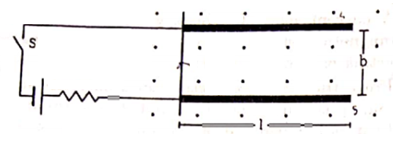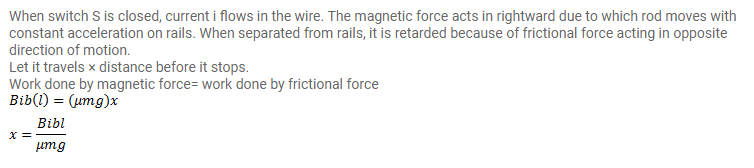# Two metal strips, each of length l,

Question:

Two metal strips, each of length l, are clamped parallel to each other on a horizontal floor with a separation b between them. A wire of mass $m$ lies on them perpendicularly as shown in figure. A vertically upward magnetic field of strength B exists in the space. The metal strips are smooth but the coefficient of friction between the wire and the floor is $\mu$. A current i is established when the switch $S$ is closed at the instant $t=0$. Discuss the motion of the wire after the switch is closed. How far away from the strips will the wire reach?Solution: